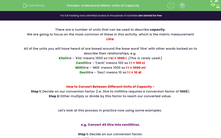# Understand Metric Units of Capacity

In this worksheet, students will practise converting into different units of measurement (involving the base word 'litre') so that they are finding and expressing equivalent capacities.Key stage:  KS 4

Year:  GCSE

GCSE Subjects:   Maths

GCSE Boards:   AQA, Eduqas, Pearson Edexcel, OCR,

Popular topics:   Geometry worksheets

Difficulty level:#### Worksheet Overview

There are a number of units that can be used to describe capacity.

We are going to focus on the most common of these in this activity, which is the metric measurement Litre.

All of the units you will have heard of are based around the base word 'litre' with other words tacked on to describe their relationships, e.g.

Kilolitre - 'Kilo' means 1000 so 1 kl = 1000 l  (This is rarely used.)

Centilitre - 'Centi' means 100 so 1 l = 100 cl

Millilitre - 'Milli' means 1000 so 1 l = 1000 ml

Decilitre - 'Deci' means 10 so 1 l = 10 dl

How to Convert Between Different Units of Capacity -

Step 1: Decide on our conversion factor (i.e. litre to millilitre requires a conversion factor of 1000);

Step 2: Either multiply or divide by this factor to reach our converted value.

Let's look at this process in practice now using some examples.

e.g. Convert 45 litre into centilitres.

Step 1: Decide on our conversion factor.

1 l = 100 cl so our conversion factor is 100.

Step 2: Multiply / divide by this factor.

One way to think about this to help make our decision is that centilitres are much smaller than litres, so there will need to be more of them overall.

To create a number which is greater than our starting total, we need to multiply

45 × 100 = 4500 cl

So 45 litres is the same as 4,500 centilitres.

Let's try another example to check we really understand this process.

e.g. Convert 750 ml to l.

Step 1:

1000 ml = 1 l so our conversion factor is 1000.

Step 2:

This time, a litre is bigger than a centilitre, so there will need to be less of them overall.

To create a number which is greater than our starting total, we need to divide: ​

750 ÷ 1000 = 0.75 l

So 750 millilitres is equivalent to 0.75 litres.

In this activity, we will convert amounts into different units of measurement (involving the base word 'litre') so that we are finding and expressing equivalent capacities, using the methods described above.

### What is EdPlace?

We're your National Curriculum aligned online education content provider helping each child succeed in English, maths and science from year 1 to GCSE. With an EdPlace account you’ll be able to track and measure progress, helping each child achieve their best. We build confidence and attainment by personalising each child’s learning at a level that suits them.

Get started